# Math > Year 2

## Tens & Ones

We use digits to make a number. Every digit in a number has a place value. To make a two-digit number, we use two digits.

Two-digit numbers are made up of tens and ones.

Objects used to show tens and ones:

Tens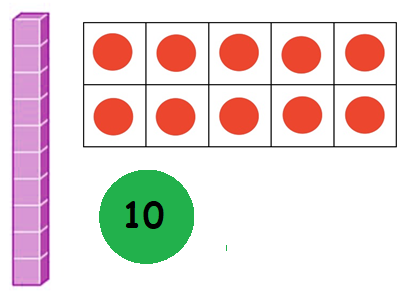OnesThe first digit tells us about tens. The second digit tells us about ones.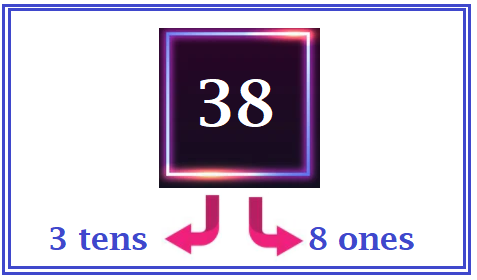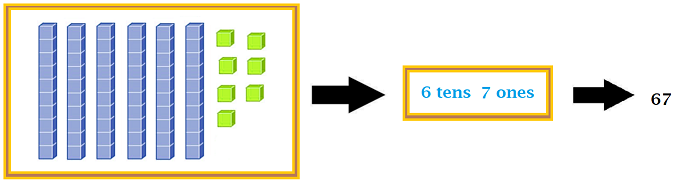Let's try some questions:

Question:

The number shown by the base blocks is _____.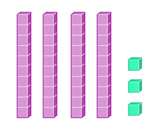1. 34

2. 304

3. 43

4. 403

Number of tens = 4

Number of ones = 3

Number shown = 43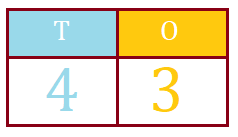Question: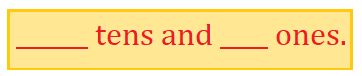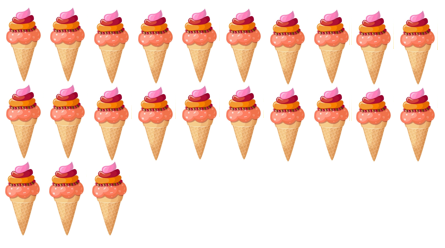1. 2 tens and 3 ones

2. 3 tens and 2 ones

3. 20 tens and 30 ones

4. 30 tens and 20 ones

Count the number of icecreams.

There are 23 icecreams.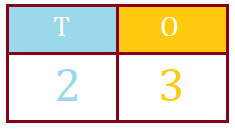There are 2 tens and 3 ones in the number 23.

Hence, option 1 is the correct answer.

//